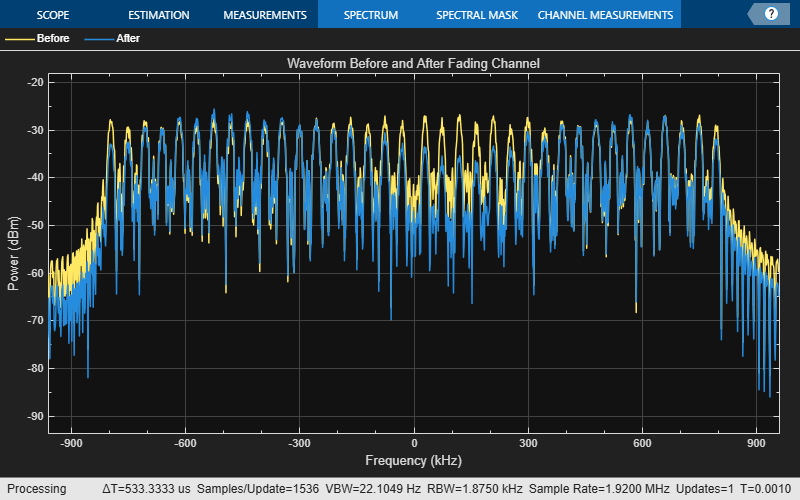# Simulate Propagation Channels

This example shows how to simulate propagation channels. It demonstrates how to generate cell-specific reference signals, map them onto a resource grid, perform OFDM modulation, and pass the result through a fading channel.

Specify the cell-wide settings as fields in the structure `enb`. Many of the functions used in this example require a subset of these fields.

```enb.NDLRB = 9; enb.CyclicPrefix = 'Normal'; enb.PHICHDuration = 'Normal'; enb.CFI = 3; enb.Ng = 'Sixth'; enb.CellRefP = 1; enb.NCellID = 10; enb.NSubframe = 0; enb.DuplexMode = 'FDD'; antennaPort = 0;```

### Resource Grid and Transmission Waveform

Generate a subframe resource grid. To create the resource grid, call the `lteDLResourceGrid` function. This function creates an empty resource grid for one subframe.

`subframe = lteDLResourceGrid(enb);`

Generate cell-specific reference symbols (CellRS) and map them onto the resource elements (REs) of a resource grid using linear indices.

```cellRSsymbols = lteCellRS(enb,antennaPort); cellRSindices = lteCellRSIndices(enb,antennaPort,{'1based'}); subframe(cellRSindices) = cellRSsymbols;```

Perform OFDM modulation of the complex symbols in a subframe, `subframe`, using cell-wide settings structure `enb`.

`[txWaveform,info] = lteOFDMModulate(enb,subframe);`

The first output argument, `txWaveform`, contains the transmitted OFDM modulated symbols. The second output argument, `info`, is a structure that contains details about the modulation process. The field `info.SamplingRate` provides the sampling rate, ${R}_{sampling}$, of the time domain waveform:

`${R}_{sampling}\phantom{\rule{0.5em}{0ex}}=\frac{30.72\phantom{\rule{0.2777777777777778em}{0ex}}MHz}{2048×{N}_{FFT}},$`

where ${N}_{FFT}$ is the size of the OFDM inverse Fourier transform (IFT).

### Propagation Channel

Construct the LTE multipath fading channel. First, set up the channel parameters by creating a structure, `channel`.

```channel.Seed = 1; channel.NRxAnts = 1; channel.DelayProfile = 'EVA'; channel.DopplerFreq = 5; channel.MIMOCorrelation = 'Low'; channel.SamplingRate = info.SamplingRate; channel.InitTime = 0;```

The sampling rate in the channel model, `channel.SamplingRate`, must be set to the `info` field of the `SamplingRate` returned by the `lteOFDMModulate` function.

Pass data through the LTE fading channel by calling the `lteFadingChannel` function. This function generates an LTE multipath fading channel, as specified in TS 36.101 (See reference ). The first input argument, `txWaveform`, is an array of LTE transmitted samples. Each row contains the waveform samples for each of the transmit antennas. These waveforms are filtered with the delay profiles as specified in the parameter structure, `channel`.

`rxWaveform = lteFadingChannel(channel,txWaveform);`

The output argument, `rxWaveform`, is the channel output signal matrix. Each row corresponds to the waveform at each of the receive antennas. Since you have defined one receive antenna, the number of rows in the `rxWaveform` matrix is one.

`size(rxWaveform)`
```ans = 1×2 1920 1 ```

### Plot Signal Before and After Fading Channel

Display a spectrum analyzer with before-channel and after-channel waveforms, using Welch's spectrum estimation method.

```title = 'Waveform Before and After Fading Channel'; saScope = spectrumAnalyzer(SampleRate=info.SamplingRate, Method='welch', ShowLegend=true,... Title = title,ChannelNames={'Before','After'}); saScope([txWaveform,rxWaveform]);```### References

1. 3GPP TS 36.101 "User Equipment (UE) radio transmission and reception".